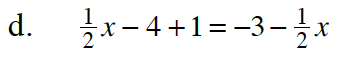Home > GB8I > Chapter cc46 > Lesson cc46.2.3 > Problem6-76

6-76.
1. Solve the following equation for x, if possible. Check your solutions. Homework Help ✎

1. −(2 − 3x) + x = 9 − x

2.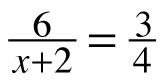3. 5 − 2(x + 6) = 14

4.x − 4 + 1 = −3 −x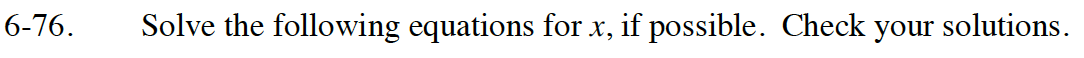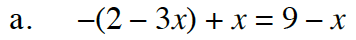Simplify the equation as much as possible, then solve for x.

x = 2.2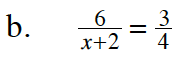Refer to part (a).

6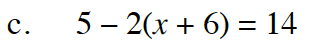Refer to part (a).